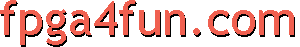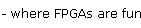## Direct Digital Synthesis 3 - Phase accumulator

#### Phase accumulator

The second DDS trick is a long phase accumulator. It allows the frequency of the signal from the DDS output to be very flexible.

We are going to see how it works with an example. Let's start with this simple code.

```reg [10:0] cnt;   // 11bit counter
always @(posedge clk) cnt <= cnt + 11'h1;

```

The counter is actually a "phase accumulator". That's because each time it increments, it moves the sine by 360°/2048=0.175°

So let's rename the counter to a better name.

```reg [10:0] phase_acc;   // 11bit
always @(posedge clk) phase_acc <= phase_acc + 11'h1;

```

Now, if we want to double the frequency of the sine output, we increment the phase accumulator by two instead of one.

```always @(posedge clk) phase_acc <= phase_acc + 11'h2;
```

But if we want to cut the frequency in half? We are out of luck as we can't increment the phase accumulator by 0.5 (Verilog supports integer numbers only). What we need is more resolution in the phase accumulator.

Let's see how it is done by adding more bits to the phase accumulator, but for now in a way that gives the same output as with the 11bit phase accumulator.

```reg [14:0] phase_acc;   // 4 more bits, for a total of 15 bits
always @(posedge clk) phase_acc <= phase_acc + 15'd16;   // increment by 16 instead of 1

```

Since we increment the counter by 16 and we use phase_acc[14:4] in the lookup address, we didn't change the output. But having four more bits gives us a phase accumulator with a better resolution. Now we can certainly decrease the output frequency in half for example (by incrementing the phase accumulator by 8 instead of 16).

Having increased the phase accumulator resolution by 16, we can have any multiple of the original sine frequency in 1/16th steps. We could certainly have added more than four bits to the phase accumulator. Typical DDS implementations use very long bits phase accumulators to have extreme precision and resolution in the output frequencies available.

For example, with a 32bit phase accumulator and a 100MHz clock, the frequency resolution of the output is 0.023Hz!
Here's a 32bit phase accumulator used to generate a 440Hz signal from a 100MHz clock.

```reg [31:0] phase_acc;   // 32bit phase accumulator
always @(posedge clk) phase_acc <= phase_acc + 18898;   // 440Hz output when clocked at 100MHz